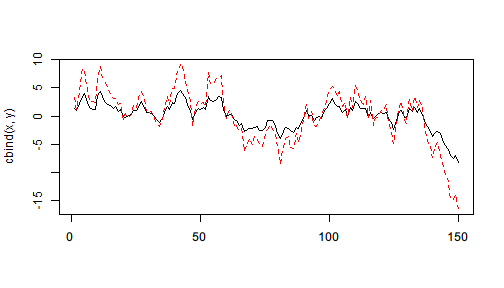# Extract element

In some cases, one might need to extract an element from an object. For example, extract r.squared from the summary of a linear model, extract the test statistics from a S4 object.

The syntax x %>>% (y) indicates to extract the element named y from object x where y must be a valid symbol name and x can be a vector, list, environment or anything else that supports [[]]. It also works with S4 object and able to extract a slot from it in exactly the same way.

mtcars %>>%
(lm(mpg ~ wt + cyl, data = .)) %>>%
summary %>>%
(r.squared)

#  0.8302274


The syntax is not ambiguous with other features that used () because evaluating a symbol simply makes no sense.

As we know that [[]] are defined for vector, list, and environment, the following simple examples work.

list(a=1,b=2) %>>% (a) # list(a=1,b=2)[["a"]]

#  1

c(a=1, b=2) %>>% (a) # c(a=1,b=2)[["a"]]

#  1


For environment,

env <- new.env()
env$a <- 1 env$b <- 2
# do more things here ...
env %>>% (a) # env$a  #  1  For S4 object, the syntax works too. We use adfTest() in library fUnitRoots to perform an ADF test to test the cointegration relationship between two vectors. Suppose the data generating process is set.seed(0) x <- cumsum(rnorm(150)) y <- 2*x + rnorm(150)*0.5 matplot(cbind(x,y),type = "l")Now given the data, we can perform an ADF test on the residues of linear model lm(y ~ x + 0). lm(y ~ x + 0) %>>% residuals %>>% fUnitRoots::adfTest(type = "nc") %>>% # S4 object (~ testobj) %>>% # save test object to environment (? class(.)) %>>% # what's the class of it? (test) %>>% # testobj@test (statistic) # testobj@test$statistic

# Warning in fUnitRoots::adfTest(., type = "nc"): p-value smaller than
# printed p-value

# ? class(.)
#  "fHTEST"
# attr(,"package")
#  "fBasics"

# Dickey-Fuller
#     -10.08887


Note that the result of fUnitRoots::adfTest() is a S4 object of class fHTEST which is assigned to testobj. The result of (test) is no longer a S4 object but an ordinary list. Therefore, there's no difference in the syntax of element extraction between S4 object and other objects that support [[]].

Since the syntax only accepts symbol name, to evaluate an expression with the piped object being the frame if it is a list or environment, using with() or within() can be helpful.

list(a = 1, b = 2) %>>%
with(a+2*b)

#  5


But this method does not work for vector and S4 object.